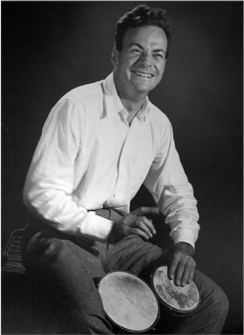Conservation of Momentum & Energy

Conservation of Momentum & Energy

Equation

W = Fd

2.2

1 Newton = 0.1 kg,

the same as a stick

of butter

What is Energy?Simply stated, energy is the capacity to do work. It exists in many forms such as thermal (heat), kinetic (mechanical), chemical, light, electrical, and potential. But what does this mean? To do work, something has to move, or more simply, change position. So, another way to define energy could be; “the ability to make things change”. Notice we are defining energy here by its observed property to induce something (work, change, etc.). So we can identify when there is energy in a system, but exactly what energy IS is very difficult to say. The famous bongo-playing physicist, Richard Feynman said:

It is important to realize that in physics today, we have no knowledge of what energy is. We do not have a picture that energy comes in little blobs of a definite amount.

What is Work?

If energy is the capacity to do work, what is work?

Work is defined as the net force times the distance that the force acts;

W = Fd

With units of newton meters or joules.

As an example, the work done in lifting a 2 kg bongo drum set from the floor ½ m to place between your legs is:

W = Fd = (2 kg x 1N____________0.1 kg) x ½ m = 20 N x ½ m = 10 Nm

Dr. Richard Feynman

Image from Brookhaven National Lab